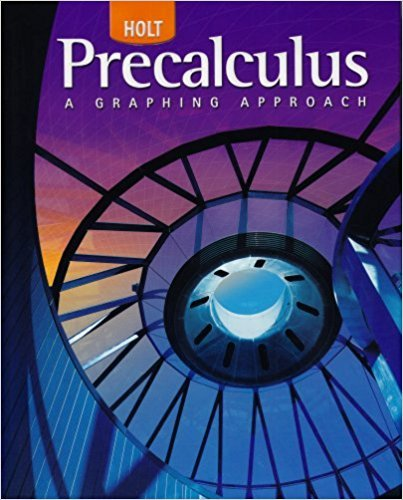×
×

# Solutions for Chapter 13.4 A: Excursion: Binomial Experiments## Full solutions for Precalculus | 1st Edition

ISBN: 9780030416477Solutions for Chapter 13.4 A: Excursion: Binomial Experiments

Solutions for Chapter 13.4 A
4 5 0 406 Reviews
21
4
##### ISBN: 9780030416477

Since 16 problems in chapter 13.4 A: Excursion: Binomial Experiments have been answered, more than 23894 students have viewed full step-by-step solutions from this chapter. Precalculus was written by and is associated to the ISBN: 9780030416477. Chapter 13.4 A: Excursion: Binomial Experiments includes 16 full step-by-step solutions. This textbook survival guide was created for the textbook: Precalculus, edition: 1. This expansive textbook survival guide covers the following chapters and their solutions.

Key Calculus Terms and definitions covered in this textbook

If u < v , then u + w < v + w

• Continuous function

A function that is continuous on its entire domain

• Course

See Bearing.

• Difference of two vectors

<u1, u2> - <v1, v2> = <u1 - v1, u2 - v2> or <u1, u2, u3> - <v1, v2, v3> = <u1 - v1, u2 - v2, u3 - v3>

• Distributive property

a(b + c) = ab + ac and related properties

• Ellipse

The set of all points in the plane such that the sum of the distances from a pair of fixed points (the foci) is a constant

• Even function

A function whose graph is symmetric about the y-axis for all x in the domain of ƒ.

• Invertible linear system

A system of n linear equations in n variables whose coefficient matrix has a nonzero determinant.

• Limit at infinity

limx: qƒ1x2 = L means that ƒ1x2 gets arbitrarily close to L as x gets arbitrarily large; lim x:- q ƒ1x2 means that gets arbitrarily close to L as gets arbitrarily large

• Mathematical induction

A process for proving that a statement is true for all natural numbers n by showing that it is true for n = 1 (the anchor) and that, if it is true for n = k, then it must be true for n = k + 1 (the inductive step)

• NDER ƒ(a)

See Numerical derivative of ƒ at x = a.

• One-to-one function

A function in which each element of the range corresponds to exactly one element in the domain

• Ordinary annuity

An annuity in which deposits are made at the same time interest is posted.

• Phase shift

See Sinusoid.

• Placebo

In an experimental study, an inactive treatment that is equivalent to the active treatment in every respect except for the factor about which an inference is to be made. Subjects in a blind experiment do not know if they have been given the active treatment or the placebo.

• Polynomial in x

An expression that can be written in the form an x n + an-1x n-1 + Á + a1x + a0, where n is a nonnegative integer, the coefficients are real numbers, and an ? 0. The degree of the polynomial is n, the leading coefficient is an, the leading term is anxn, and the constant term is a0. (The number 0 is the zero polynomial)

• Reflection across the x-axis

x, y and (x,-y) are reflections of each other across the x-axis.

• Terms of a sequence

The range elements of a sequence.

• Upper bound test for real zeros

A test for finding an upper bound for the real zeros of a polynomial.

• xz-plane

The points x, 0, z in Cartesian space.

×